§4     数论函数

对任一正整数n有确定值的函数f(n)称为数论函数.

[积性函数与完全积性函数]  (m,n)=1,f(mn)=f(m)f(n),则称数论函数f(n)为积性函数.若对任意正整数m,n都有f(mn)=f(m)f(n),则称f(n)为完全积性函数.

积性函数具有下列性质:

1°  f(n)为非零积性函数,f(1)=1.

2°  g(n),h(n)都为积性函数,g(n)h(n)仍为积性函数.3°  g(n)为非零积性函数,,4°  f(n)为积性函数,

f([m,n])f((m,n))=f(m)f(n)

[麦比乌斯函数]  函数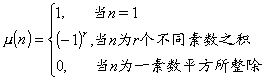麦比乌斯函数具有下列性质:

1°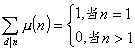2°  μ(n)为积性函数,但非完全积性函数.

3°,f(n)为积性函数,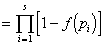`也为积性函数.例如`
``
``
`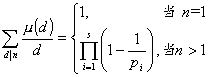`[欧拉函数]  n为自然数,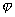(n)为不超过n且与n互素的正整数的个数,称为欧拉函数.

欧拉函数具有下列性质:

1°(n)为积性函数,但非完全积性函数.

2°,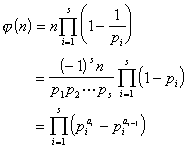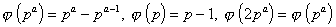3°4°[除数函数]  自然数n的全部因数的个数称为除数函数,记作d(n).除数函数具有下列性质:

1°  d(n) 为积性函数,但非完全积性函数,对任意自然数m,n,常有2°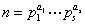,[冯・曼哥特函数]  函数

L (n)[麦比乌斯反转公式与麦比乌斯变换]

1°  反转公式一,又设h(k)为一非零完全积性函数.若对所有适合于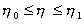h常有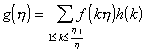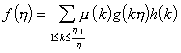2°  反转公式二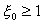,又设H(k)为一非零完全积性函数.若对所有适合于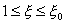x 常有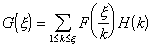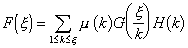3°  反转公式三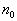为一正整数, 又设h(k)为一非零完全积性函数.若对所有常有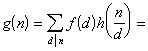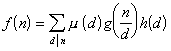4°  麦比乌斯变换  n为正整数,g(n)称为f(n)的麦比乌斯变换,f(n)称为g(n)的麦比乌斯逆变换.

5°  乘积麦比乌斯变换  n为正整数,g(n)称为f(n)的乘积麦比乌斯变换,f(n)称为g(n)的乘积麦比乌斯逆变换.

[麦比乌斯变换表]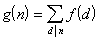g(n) f(n)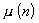d(n)1d(n) 1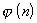n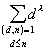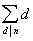n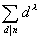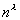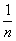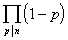L (n) -logn logn L (n)